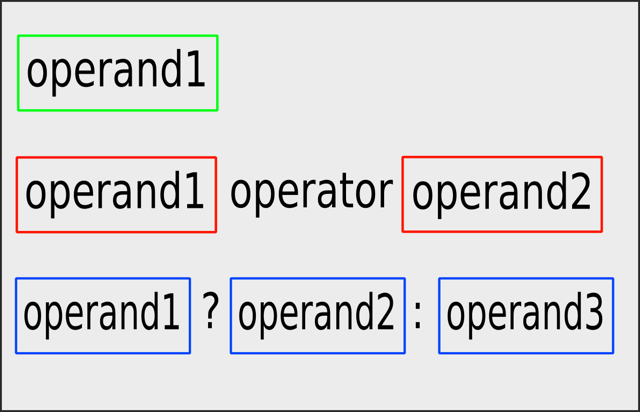## 公告

《如何自學程式設計》入選 Google Play 台灣地區2016年度最佳書籍｜本站於 Google Play 銷售電子書。

------
Udemy ｜ Python 專案開發入門的二十一堂課 | 促銷優惠

### C++ 入門指南 - 運算式與陳述```a; // 以變數當運算元
3; // 以字面常數當運算元
printPrompt(); // 呼叫 printPrompt() 當運算元```

++a
--a
++遞增++a, a++
--遞減--a, a--
!邏輯補數運算子~a

```int a = -3;
int b = +a; // b 會等於 -3
int c = -a; // c 會等於 3```

```int a = 3;
int b = ++a; // b 會等於 4
int c = a++; // c 會等於 3
int d = --a; // b 會等於 2
int e = a--; // e 會等於 3```

```bool a = true;
bool b = !a; // b 會等於 false
bool c = !b; // c 會等於 true```

+a + b
-a - b
*a * b
/a / b
%取餘數a % b

```int a = 11;
int b = a + 33; // b 會等於 44
int c = b - 22; // c 會等於 22
int d = c * 11; // d 會等於 242
int e = d / 44; // e 會等於 5
int f = e % 55; // f 會等於 5```

==相等a == b
!=不相等a != b
>大於a >= b
>=大於等於a >= b
<小於a < b
<=小於等於a <= b

```int a = 11;
int b = 22;

bool c = a >= b; // c 會等於 false
bool d = a <= b; // d 會等於 true
bool e = c == d; // e 會等於 false
bool f = a != b; // f 會等於 true```

=指派a = b
+=相加同時指派a += b
-=相減同時指派a -= b
*=相乘同時指派a *= b
/=相除同時指派a /= b
%=取餘數同時指派a %= b

```int a = 11;
a += 33; // a 會等於 44
a -= 22; // a 會等於 22
a *= 11; // a 會等於 242
a /= 44; // a 會等於 5
a %= 55; // a 會等於 5```

`f = (a != b); // 等於 f = a != b`

`; // 什麼事也不做的陳述`

http://www.cplusplus.com/doc/tutorial/operators/